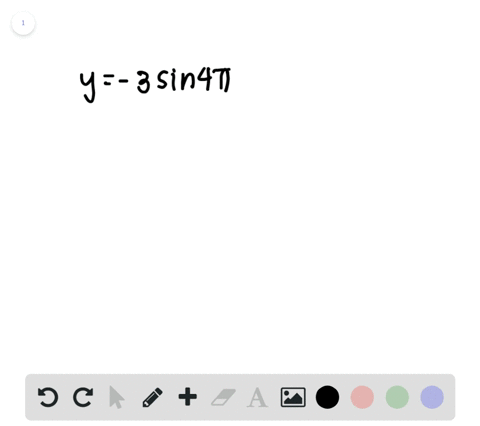🎉 The Study-to-Win Winning Ticket number has been announced! Go to your Tickets dashboard to see if you won! 🎉View Winning Ticket### Period and Amplitude In Exercises $45-48,$ determ…

00:45University of Southern California
Problem 46

# Period and Amplitude In Exercises $45-48,$ determine the period and amplitude of each function.$$y=\frac{3}{2} \cos \frac{x}{2}$$

## Discussion

You must be signed in to discuss.

## Video Transcript

problem. Number 46 asks us to determine the period and amplitude of the following function. Why equals three half's co sign of uh, X, divided by two Now for any co sign function in the form, Why equals a co sign of B X? We know that the period is always going to equal be divided by oops. Excuse me for that. It's always gonna equal to pi, divided by the absolute value of being. And in this instance, B equals 1/2. So our period, he's gonna be two pi divided by the absolute value of 1/2. 1/2 is positive so we can drop that absolute value bar. And since we're dividing by a fraction, we can multiply by. It's reciprocal. So we have two times too high equals four pi. So the period of this function equals four part now onto the amplitude, which is the easier part. The amplitude always equals a and in this instance, a equals three house. So our amplitude equals three halves. And after we find these values, you could come over here and look at our function and see that that makes about sense. Um, if we look here the amplitude seems to be about one in what half. So this distance right here is three halfs and 112 334 This looks to be about four pi.